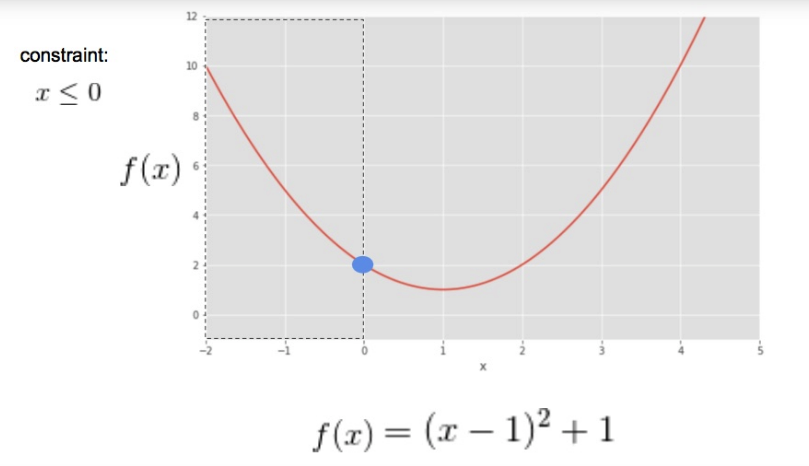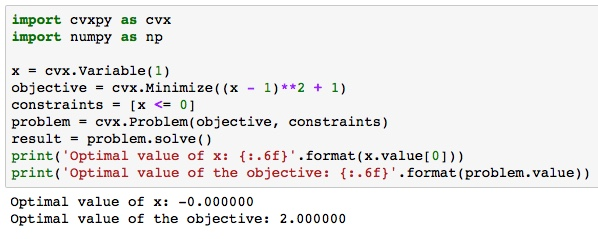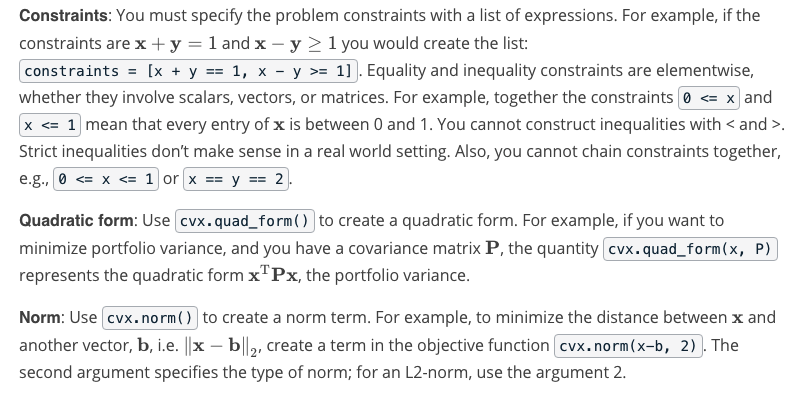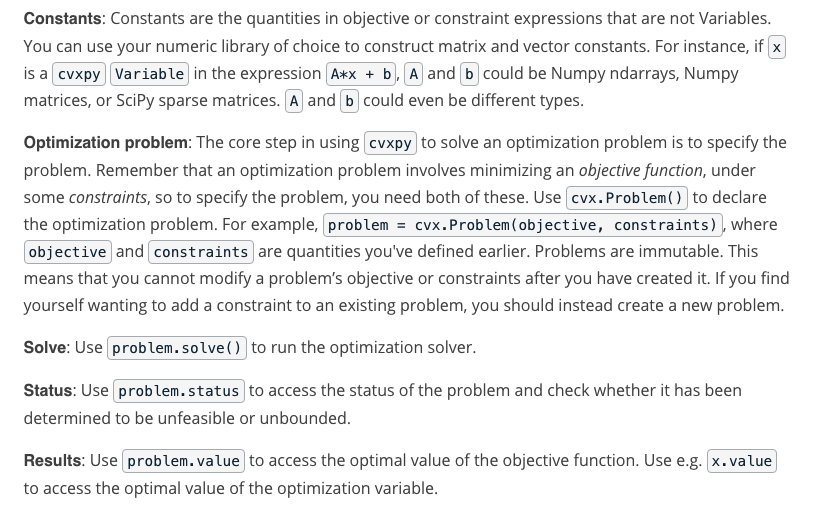## cvxpy

What is cvxpy? cvxpy is a Python package for solving convex optimization problems. It allows you to express the problem in a human-readable way, calls a solver, and unpacks the results.

cvxpy 什么是cvxpy？ cvxpy是一个用于解决凸优化问题的Python包。它允许您以人类可读的方式表达问题，调用解算器并解压缩结果。

## cvxpy Example

Below, please find an example of the use of cvxpy to solve the simple example we referred to in the lectures, optimizing the objective function$(x - 1)^2 +1$ subject to the constraint x≤0.## How to use cvxpy

Import: First, you need to import the package:import cvxpy as cvx

Steps: Optimization problems involve finding the values of a variable that minimize an objective function under a set of constraints on the range of possible values the variable can take. So we need to use cvxpy to declare the variable, objective function and constraints, and then solve the problem.

Optimization variable: Use cvx.Variable() to declare an optimization variable. For portfolio optimization, this will be x, the vector of weights on the assets. Use the argument to declare the size of the variable; e.g. x = cvx.Variable(2) declares that x is a vector of length 2. In general, variables can be scalars, vectors, or matrices.

Objective function: Use cvx.Minimize() to declare the objective function. For example, if the objective function is $(\mathbf{x} - \mathbf{y})^2$ , you would declare it to be:objective = cvx.Minimize((x - y)**2).## Exercise:cvxpy

Portfolio Optimization using cvxpy

## Install cvxpy and other libraries

import sys
!{sys.executable} -m pip install -r requirements.txt
Requirement already satisfied: colour==0.1.5 in /opt/conda/lib/python3.6/site-packages (from -r requirements.txt (line 1))
Collecting cvxpy==1.0.3 (from -r requirements.txt (line 2))
[K    100% |████████████████████████████████| 880kB 517kB/s eta 0:00:01
[?25hRequirement already satisfied: cycler==0.10.0 in /opt/conda/lib/python3.6/site-packages/cycler-0.10.0-py3.6.egg (from -r requirements.txt (line 3))
Collecting numpy==1.14.5 (from -r requirements.txt (line 4))

[33mYou are using pip version 9.0.1, however version 19.0.3 is available.
You should consider upgrading via the 'pip install --upgrade pip' command.[0m

## Imports

import cvxpy as cvx
import numpy as np
import quiz_tests

## Optimization with cvxpy

http://www.cvxpy.org/

Practice using cvxpy to solve a simple optimization problem. Find the optimal weights on a two-asset portfolio given the variance of Stock A, the variance of Stock B, and the correlation between Stocks A and B. Create a function that takes in these values as arguments and returns the vector of optimal weights, i.e.,

$\mathbf{x} = \begin{bmatrix} x_A & x_B \end{bmatrix}$

Remember that the constraint in this problem is: $x_A + x_B = 1$

## Hints

### standard deviation

standard deviation $\sigma_A = \sqrt(\sigma^2_A)$, where $\sigma^2_A$ is variance of $x_A$
look at np.sqrt()

### covariance

correlation between the stocks is $\rho_{A,B}$

covariance between the stocks is $\sigma_{A,B} = \sigma_A \times \sigmaB \times \rho{A,B}$

### x vector

create a vector of 2 x variables $\mathbf{x} = \begin{bmatrix} x_A & x_B \end{bmatrix}$
we can use cvx.Variable(2)

### covariance matrix

The covariance matrix $P = \begin{bmatrix} \sigma^2A & \sigma{A,B} \ \sigma_{A,B} & \sigma^2_B \end{bmatrix}$

We can create a 2 x 2 matrix using a 2-dimensional numpy array
np.array([["Cindy", "Liz"],["Eddy", "Brok"]])

We can write the portfolio variance $\sigma^2_p = \mathbf{x^T} \mathbf{P} \mathbf{x}$

Recall that the $\mathbf{x^T} \mathbf{P} \mathbf{x}$ is called the quadratic form.
We can use the cvxpy function quad_form(x,P) to get the quadratic form.

### objective function

Next, we want to define the objective function. In this case, we want to minimize something. What do we want to minimize in this case? We want to minimize the portfolio variance, which is defined by our quadratic form $\mathbf{x^T} \mathbf{P} \mathbf{x}$

We can find the objective function using cvxpy objective = cvx.Minimize(). Can you guess what to pass into this function?

### constraints

We can also define our constraints in a list. For example, if you wanted the $\sum_{1}^{n}x = 1$, you could save a variable as [sum(x)==1], where x was created using cvx.Variable().

### optimization

So now that we have our objective function and constraints, we can solve for the values of $\mathbf{x}$.
cvxpy has the constructor Problem(objective, constraints), which returns a Problem object.

The Problem object has a function solve(), which returns the minimum of the solution. In this case, this is the minimum variance of the portfolio.

It also updates the vector $\mathbf{x}$.

We can check out the values of $x_A$ and $x_B$ that gave the minimum portfolio variance by using x.value

import cvxpy as cvx
import numpy as np

def optimize_twoasset_portfolio(varA, varB, rAB):
"""Create a function that takes in the variance of Stock A, the variance of
Stock B, and the correlation between Stocks A and B as arguments and returns
the vector of optimal weights

Parameters
----------
varA : float
The variance of Stock A.

varB : float
The variance of Stock B.

rAB : float
The correlation between Stocks A and B.

Returns
-------
x : np.ndarray
A 2-element numpy ndarray containing the weights on Stocks A and B,
[x_A, x_B], that minimize the portfolio variance.

"""
# TODO: Use cvxpy to determine the weights on the assets in a 2-asset
# portfolio that minimize portfolio variance.

cov = np.sqrt(varA)*np.sqrt(varB)*rAB

x = cvx.Variable(2)

P = np.array([[varA, cov],[cov, varB]])

constraints = [sum(x)==1]

problem = cvx.Problem(objective, constraints)

min_value = problem.solve()
xA,xB = x.value

# return xA and xB
return xA, xB

quiz_tests.test_optimize_twoasset_portfolio(optimize_twoasset_portfolio)
Tests Passed
"""Test run optimize_twoasset_portfolio()."""
xA,xB = optimize_twoasset_portfolio(0.1, 0.05, 0.25)
print("Weight on Stock A: {:.6f}".format(xA))
print("Weight on Stock B: {:.6f}".format(xB))
Weight on Stock A: 0.281935
Weight on Stock B: 0.718065